1、先通过对N个训练样本的学习得到第一个弱分类器； 2、将分错的样本和其他的新数据一起构成一个新的N个的训练样本，通过对这个样本的学习得到第二个弱分类器； 3、将1和2都分错了的样本加上其他的新样本构成另一个新的N个的训练样本，通过对这个样本的学习得到第三个弱分类器 4、最终经过提升的强分类器。即某个数据被分为哪一类要由各分类器权值决定。

1. 使用加权后选取的训练数据代替随机选取的训练样本，这样将训练的焦点集中在比较难分的训练数据样本上； 　　 2. 将弱分类器联合起来，使用加权的投票机制代替平均投票机制。让分类效果好的弱分类器具有较大的权重，而分类效果差的分类器具有较小的权重。

1. 给定训练样本集S，其中X和Y分别对应于正例样本和负例样本；T为训练的最大循环次数； 2. 初始化样本权重为1/n ，即为训练样本的初始概率分布； 　　 3. 第一次迭代：(1)训练样本的概率分布相当，训练弱分类器;(2)计算弱分类器的错误率;(3)选取合适阈值，使得误差最小；(4)更新样本权重； 　　 经T次循环后，得到T个弱分类器，按更新的权重叠加，最终得到的强分类器。

``````Given: m examples (x1, y1), …, (xm, ym)
where xiX, yiY={-1, +1}
xi表示X中第i个元素，
yi表示与xi对应元素的属性值，+1表示xi属于某个分类，
-1表示xi不属于某个分类
``````

``````xi的权重D(i) :i=1,……，m;
(1).若正负样本数目一致，D1(i) = 1/m
(2).若正负样本数目m+, m-则正样本D1(i) = 1/m+，
负样本D1(i) = 1/m-
``````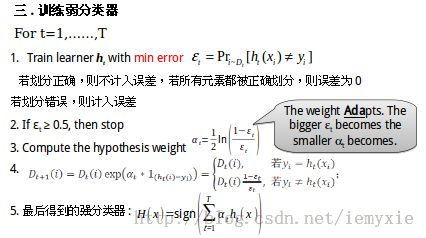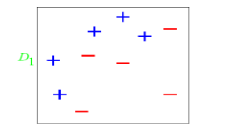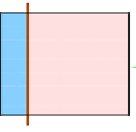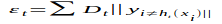计算可得`e1=（0.1+0.1+0.1）/1.0=0.3`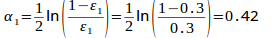``````D1=D0*(1-e1)/e1=0.1*(1-0.3)/0.3=0.2333
``````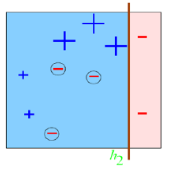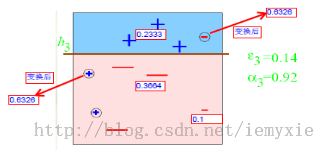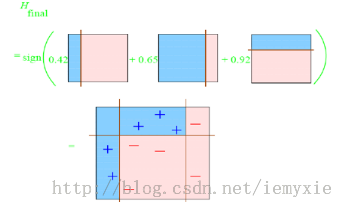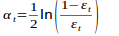1)容易受到噪声干扰，这也是大部分算法的缺点

2)训练时间过长

3)执行效果依赖于弱分类器的选择

SQL实现

``````#开始迭代
while(@i<=3) do
set @evalue=0,@temp=0;
set @flag1=0,@flag2=0,@flag3=0,@flag4=0;
set @las=concat('d',@i-1);
set @cur=concat('d',@i);
set @a=concat('select hx,hy into @hx,@hy from hea where id = ',@i);
prepare stmt1 from @a;
execute stmt1;
set @aa=concat('update adaset set ',@cur,' = ',@las);
prepare stmt111 from @aa;
execute stmt111;
#1.分类器为垂直x轴直线
if (@hy=0) then
#处理分类1
set @b=concat('select count(class) into @l_1 from adaset where class=1 and x < ',@hx);
prepare stmt2 from @b;
execute stmt2;
set @c=concat('select count(class) into @l_2 from adaset where class=-1 and x < ',@hx);
prepare stmt3 from @c;
execute stmt3;
if(@l_1=0 and @l_2!=0) then
set @clas=concat('update hea set l=-1 where id = ',@i);
prepare stmt28 from @clas;
execute stmt28;
end if;
if(@l_1!=0 and @l_2 =0) then
set @clas=concat('update hea set l=1 where id = ',@i);
prepare stmt29 from @clas;
execute stmt29;
end if;
set @weight=concat('d',@i-1);
if (@l_1 !=0 and @l_2 !=0 and @l_1>@l_2) then #@l_2是错分点
set @d=concat('select sum(',@weight,') into @temp from adaset where class=-1 and x < ',@hx);
prepare stmt4 from @d;
execute stmt4;
set @[email protected][email protected];
set @flag1=1;
set @clas=concat('update hea set l=1 where id = ',@i);
prepare stmt20 from @clas;
execute stmt20;
end if;
if (@l_1 !=0 and @l_2 !=0 and @l_1<@l_2) then #@l_1是错分点
set @d=concat('select sum(',@weight,') into @temp from adaset where class=1 and x < ',@hx);
prepare stmt5 from @d;
execute stmt5;
set @[email protected][email protected];
set @flag2=1;
set @clas=concat('update hea set l=-1 where id = ',@i);
prepare stmt21 from @clas;
execute stmt21;
end if;
#总权值&误差
set @h=concat('select sum(',@weight,') into @temp from adaset');
prepare stmt10 from @h;
execute stmt10;
set @evalue = round(@evalue/@temp,4);
set @avalue = round((0.5*ln(([email protected])/@evalue)),4);
set @eee=round(([email protected])/@evalue,4);
#更新误差e&假设权重a
set @j=concat('update hea set e = ',@evalue,' ,a = ',@avalue,' where id = ',@i);
prepare stmt11 from @j;
execute stmt11;
#更新错分样本的权重
if (@hy=0) then
if (@flag1=1) then
set @k=concat('update adaset set ',@cur,' = ',@las,'*',@eee,' where class=-1 and x < ',@hx);
prepare stmt12 from @k;
execute stmt12;
end if;
if (@flag2=1) then
set @m=concat('update adaset set ',@cur,' = ',@las,'*',@eee,' where class=1 and x < ',@hx);
prepare stmt13 from @m;
execute stmt13;
end if;
if (@flag3=1) then
set @n=concat('update adaset set ',@cur,' = ',@las,'*',@eee,' where class=-1 and x > ',@hx);
prepare stmt14 from @n;
execute stmt14;
end if;
if (@flag4=1) then
set @o=concat('update adaset set ',@cur,' = ',@las,'*',@eee,' where class=1 and x > ',@hx);
prepare stmt15 from @o;
execute stmt15;
end if;
end if;
set @[email protected]+1;
end while;
``````